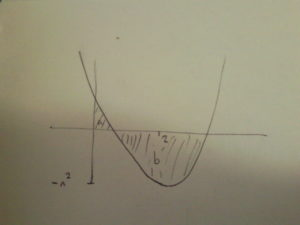I was sent this by my friend and colleague TeaKay from Blogstronomy, and I’ve adapted the puzzle slightly to make the sums a little bit nicer.

A curve has the equation $y = (x-2)^2 - n^2$. The area bounded by the co-ordinate axes and the curve in the first quadrant is equal to the area bounded by the $x$-axis and the curve in the fourth quadrant. What is the value of $n$?The puzzle boils down to working out the areas marked $a$ and $b$ in the picture - which is an integration problem. The question doesn’t give us limits, though! Luckily, we can work them out.

Area $a$ will have limits from 0 to where the curve first crosses the axis; area $b$ will have limits from the first solution to the second. And where are the solutions? Where $y = 0$, of course.

$(x-2)^2 - n^2 = 0$ $(x-2)^2 = n^2$ $x-2 = \pm n$ $x = 2 ± n$.

The first solution is at $x=2-n$; the second is at $x=2+n$.

How about the integral? Let’s do it without limits for the moment, using a neat trick from C3 - you can integrate a simple bracket with just one x in it without multiplying it out: $I = \int ((x-2)^2 - n^2) \dx = \frac{1}{3}(x-2)^3 - x n^2 + c$

When $x = 2-n$, you get: $I = -\frac{1}{3} n^3 - (2-n) n^2 + c = -\frac{1}{3}n^3 - 2n^2 + n^3 + c = \frac{2}{3}n^3 - 2n^2 + c.$

When $x = 0$, you get: $I = \frac{1}{3}(-2)^3+ c = -\frac{8}{3} + c$

So the integral from 0 to 2-n gives $a = \frac{2}{3}n^3 - 2n^2 + \frac{8}{3}.$

When $x = 2+n$, you get: $I = \frac{1}{3}n^3 - (2+n)n^2 + c = \frac{1}{3} n^3 - 2n^2 - n^3 + c$ $= -\frac{2}{3}n^3 - 2n^2 + c.$

That means the integral from $x = 2-n$ to $x = 2+n$ gives: $(-\frac{2}{3}n^3 - 2n^2 + c) - (\frac{2}{3}n^3 - 2n^2 + c) = -\frac{4}{3}n^3$.

It’s negative because it’s below the axis, so $b = \frac{4}{3}n^3$.

Now we’ve got $a = b$, so $\frac{2}{3}n^3 - 2n^2 + \frac{8}{3} = \frac{4}{3}n^3.$

First up, let’s treble everything to get rid of the fractions: $2n^3 - 6n^2 + 8 = 4n^3$

And rearrange: $0 = 2n^3 + 6n^2 - 8$ $0 = n^3 + 3n^2 - 4.$

How do you solve a cubic? Well, one way is to guess or, as the mathematicians call it, ‘solve by inspection’. It doesn’t take much thinking to spot that $n = 1$ is a solution to that equation.

You can do long division on it to see if it has other solutions: $(n^3 + 3n^2 - 4) ÷ (n - 1) = n^2 + 4n + 4 = (n+2)^2.$

So the three solutions are $n = 1$ and $n = -2$, twice. However, $n = -2$ doesn’t fit the diagram because the solutions would be at 0 and 4.

So we’re done! $n = 1$ is the only solution that makes sense.

* Edited 2016-02-11 to make it all LaTeX and fancy. * Edited 2016-02-13 after @realityminus3 spotted an error. Thanks!